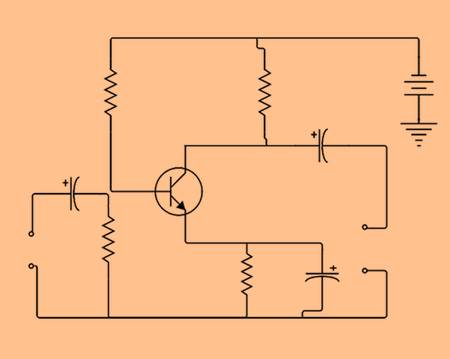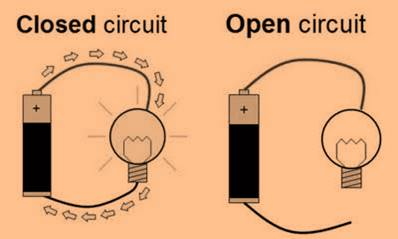## Circuit diagram

• When an electric circuit is represented in a convection pictorial form using symbols for Electronic components, then it is called a circuit diagram.
• It is also known as wiring diagram, elementary diagram, electrical diagram or electronic schematic.## Electric circuit

• An electric circuit is a closed path created by the connection of different electronic components by which current flows.
• A battery should be connected if a circuit needs more power.

### Open Circuit

• When through a circuit current does not flow then it is called open circuit.
• Its switch has an off position.

### Closed circuit

• When through a circuit current flows then it is called open circuit.
• Its switch has an on position.### The heating effect of electric current

• When through the filament of the bulb, an electric current flows then heat gets generated and bulb becomes hot.
• This property is called a heating effect of an electric current.

### Heating elements

• Heating elements have wire coils which produce heat.
• The function of the appliance decides the type of heating element.

### The magnetic effect of electric current

• The bar acts like a magnet when around an iron bar electric current runs through a wire.
• This particular magnet is named as an electromagnet.
• Hans Christian Oersted discovered this effect.
• Two pieces of insulated copper, a battery, a bulb, a nail, and paper clips of iron are required to make an electromagnet

### Application of an electromagnet

• Toys
• Medicines
• Electric bell
• Iron industries## Recap

• When an electric circuit is represented in a convection pictorial form using symbols for Electronic components, then it is called a circuit diagram.
• A battery should be connected if a circuit needs more power.
• When through a circuit current does not flow then it is called open circuit.
• When through a circuit current flows then it is called open circuit.
• The bar acts like a magnet when around an iron bar electric current runs through a wire.